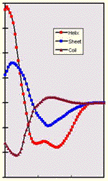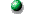# BR's crystallographic computing tutorialsImplementation of CD spectra fit

The problem is quite straight forward, namely to read data, baseline, standard curves and some experimental details and list the results of the fit in a format which can be plotted easily using any spreadsheet program. The program checks for proper range of data, applies a baseline correction if needed, and attempts to solve the fit by a fast general LSQ method. If that procedure returns a negative coefficient, the program imposes positivity on the problem by iteratively hacking through all possible combinations and flags the best one. Final output is a listing of statistical parameters, data lists and a spline to the data, just for exercise.

## Code

First, the data, baseline, standard and header files are read and the overlapping wavelength range determined. After reading the files, finding the proper range of data, and calculating the conversion factors from sample ellipticity to mean residual ellipticity in [deg.cm2/(dmole.residue)] we put out the header:

```c --- knock out header                                                  CDFI0200
rres=float(ires)                                                  CDFI0201
write (8,'(a)') ' -----------------------------------------------'CDFI0202
write (8,'(a)') ' CD DATA fitted by CD-FIT program (B.Rupp, 1996)'CDFI0203
write (8,'(a)') ' -----------------------------------------------'CDFI0204
write(8,'(a,a)')  ' CD raw data file        : ',datfil            CDFI0205
write(8,'(a,a)')  ' CD baseline file        : ',basfil            CDFI0206
write(8,'(a,a)')  ' Sample header file      : ',hedfil            CDFI0207
write(8,'(a,a)')  ' Ellipticity output file : ',outfil            CDFI0208
write(8,'(a,a)')  ' Results file            : ',resfil            CDFI0209
write(8,'(a,a/)') ' Standard data file      : ',lysfil            CDFI0210
write(8,'(i3,a,i3,a,i3,a)') npts,' data points from ',            CDFI0211
& ilo,' to ',ihi,' nm'                                             CDFI0212
write(8,*)         '--------------------------------------------' CDFI0213
write(8,'(a,f10.2)') ' Molecular weight       g/mol   :  ',mw     CDFI0214
write(8,'(a,f10.6)') ' Sample concentration  mg/cm3   :  ',cconc  CDFI0215
write(8,'(a,f10.8)') ' Sample concentration   g/cm3   :  ',conc   CDFI0216
write(8,'(a,f10.8)') ' Sample concentration mol/cm3   :  ',cmol   CDFI0217
write(8,'(a,f10.6)') ' Cell path             mm       :  ',ppath  CDFI0218
write(8,'(a,f10.6)') ' Cell path             cm       :  ',path   CDFI0219
write(8,'(a,f10.6)') ' Number of residues             :  ',rres   CDFI0220
CDFI0240
c --- in deg*cm2/dmol                                                   CDFI0241
dfact=mw/(10.0*conc*path)                                         CDFI0242
write(8,'(a,f12.2)') ' Conversion factor [cm2/dmole]  :',         CDFI0244
&   dfact                                                          CDFI0245
write(8,*)        '---------------------------------------------' CDFI0246```

We perform baseline subtraction and a final baseline correction assuming that, based on the model we are using, the signal between 245 and 250 nm should be zero.

```c --- create the CD data from bas and knp and 18/pi                     CDFI0252
itest=0                                                           CDFI0253
do i=ilo,ihi                                                      CDFI0254
c ---    mean residual ellipticty                                       CDFI0256
elli(i)=cd(i)*dfact/ires                                       CDFI0257
if(itest.eq.1) write(*,*) i,cd(i),elli(i)                      CDFI0258
end do                                                            CDFI0259
CDFI0260
c --- create zero shift only if sufficient data available               CDFI0261
if (ihi.ge.250) then                                              CDFI0262
iavg=ihi-245                                                   CDFI0263
do j=250-iavg,250                                              CDFI0264
sumbas=sumbas+elli(j)                                       CDFI0265
end do                                                         CDFI0266
sumbas=sumbas/(iavg+1)                                         CDFI0267
do j=ilo,ihi                                                   CDFI0268
elli(j)=elli(j)-sumbas                                      CDFI0269
end do                                                         CDFI0270
write (*,'(a,f5.0)') ' Zero shift applied : ',sumbas           CDFI0271
write (8,'(1x,a,f5.0)') ' Zero shift applied : ',sumbas        CDFI0272
else                                                              CDFI0273
sumbas=0.0                                                     CDFI0274
write(*,*)'Cannot determine zero shift for given data range'   CDFI0275
write(8,'(a)')'Cannot determine zero shift for data range'     CDFI0276
end if                                                            CDFI0277```

Now we are ready to set up the normal equations by filling the coefficient matrix A with the corresponding sums and call the Gauss-Jordan elimination to solve the equations:

```c --- calculate fit by LSQ procedure -----------------------------------CDFI0279
c                                                                       CDFI0280
c      A.x = b where A LSQ matrix                                       CDFI0281
c      j : data points (1=helix,2=sheet,3=coil) at lambda, sum is over  CDFI0282
c      all used lambda's, m=measured value at lamba                     CDFI0283
c      A(i,j)=sum(i,j) 3x3                                              CDFI0284
c      b(i)=sum(m*j)   1x3                                              CDFI0285
c                                                                       CDFI0286
c ----------------------------------------------------------------------CDFI0287
a=0                                                               CDFI0288
b=0                                                               CDFI0289
x=0                                                               CDFI0290
datapt(1,ilo:ihi)=helix(ilo:ihi)                                  CDFI0291
datapt(2,ilo:ihi)=sheet(ilo:ihi)                                  CDFI0292
datapt(3,ilo:ihi)=coil(ilo:ihi)                                   CDFI0293
c --- fill normal equations                                             CDFI0294
do i=1,3                                                          CDFI0295
do k=ilo,ihi                                                   CDFI0296
b(i)=b(i)+elli(k)*datapt(i,k)                               CDFI0297
end do                                                         CDFI0298
do j=1,3                                                       CDFI0299
do k=ilo,ihi                                              CDFI0300
a(i,j)=a(i,j)+datapt(i,k)*datapt(j,k)                    CDFI0301
end do                                                      CDFI0302
end do                                                         CDFI0303
end do                                                            CDFI0304
call gaussj(a,3,3,b,1,1)                                          CDFI0305```

Now we have to make sure that the sum of the components are 100% of the measured signal. As experimental errors as well as simplified model assumptions usually give less than 100% for the sum of the three components. If the result is really off, there might be a problem with the data, or the fit by sum of ideal helix, sheet and coil is questionable or inappropriate.

```c --- check results and normalize                                       CDFI0306
scfac=0                                                           CDFI0307
ifit=0                                                            CDFI0308
do i=1,3                                                          CDFI0309
if (b(i).lt.0) then                                            CDFI0310
ifit=1                                                      CDFI0311
else                                                           CDFI0312
scfac=scfac+b(i)                                            CDFI0313
end if                                                         CDFI0314
end do                                                            CDFI0315
b=b*100                                                           CDFI0316
write(*,'(/a)')' Least squares percentage(s) and scale factor'    CDFI0317
write(*,'(4f10.2)') b(1)/scfac,b(2)/scfac,b(3)/scfac,scfac        CDFI0318
write(8,'(/a)')' Least squares percentage(s) and scale factor'    CDFI0319
write(8,'(4f10.2)') b(1)/scfac,b(2)/scfac,b(3)/scfac,scfac        CDFI0320```

We need to check for another problem resulting from the oversimplified model : One of the fit parameters can be negative and the solution is physically not meaningful (see LSQ tutorial for other means to constrain the solutions). We then resume a brute force calculation of every possible combination of helix coil and sheet and check which one is best.

Let's think about this a little : if we consider 2% accurate enough for a CD fit (rest assured that this is a rather optimistic assumption) we need to loop over 50*50*50 summations of all datapoints, about 60 here. Compare the LSQ solution : all that was needed was a 3 times loop over the datapoints to sum up the LSQ matrix:. The iteration therefore needs about 42000 times more operations than the LSQ refinement. Not an option for any problem of complexity, but still feasable in this case.

```      if (ifit) then                                                    CDFI0322
write (*,'(a)')' Negative LSQ parameters - cannot solve by LSQ'CDFI0323
write (*,'(a/)')' Will resume with iterative procedure '       CDFI0324
write (8,'(a)')' Negative LSQ parameters - cannot solve by LSQ'CDFI0325
write (8,'(a/)')' Will resume with iterative procedure '       CDFI0326
c ---    calculate fit by iteration ---                                 CDFI0327
srs=1.0E10                                                     CDFI0328
aa=0                                                           CDFI0329
bb=0                                                           CDFI0330
cc=0                                                           CDFI0331
iprec=2                                                        CDFI0332
write(*,'(a\$)')' Fitting'                                      CDFI0333
do i=0,100,iprec                                               CDFI0334
write(*,'(a\$)')'.'                                          CDFI0335
do j=0,100,iprec                                            CDFI0336
do k=0,100,iprec                                         CDFI0337
srs2=0                                                CDFI0338
do m=ilo,ihi                                          CDFI0339
difa=elli(m)-0.01*(i*helix(m)+j*sheet(m)+k*coil(m))CDFI0340
srs2=srs2+difa**2                                  CDFI0341
end do                                                CDFI0342
srs2=sqrt(srs2)                                       CDFI0343
if (srs2.lt.srs) then                                 CDFI0344
srs=srs2                                           CDFI0345
aa=i                                               CDFI0346
bb=j                                               CDFI0347
cc=k                                               CDFI0348
end if                                                CDFI0349
end do                                                   CDFI0350
end do                                                      CDFI0351
end do                                                         CDFI0352
scfac=(aa+bb+cc)/100                                           CDFI0353
else                                                              CDFI0354
aa=b(1)                                                        CDFI0355
bb=b(2)                                                        CDFI0356
cc=b(3)                                                        CDFI0357
end if                                                            CDFI0358
write (*,'(/a)') ' '                                              CDFI0359```

Finally, we compute the r.m.s.d. of the fit and a R-value and print out the results, statistics, and fitted datat.

```c --- compute the data values and differences for best fit              CDFI0361
sdif=0                                                            CDFI0362
sobs=0                                                            CDFI0363
srs=0                                                             CDFI0364
do j=ilo,ihi                                                      CDFI0365
cdft(j)=0.01*(aa*helix(j)+bb*sheet(j)+cc*coil(j))              CDFI0366
dif(j)=elli(j)-cdft(j)                                         CDFI0367
sdif=sdif+abs(dif(j))                                          CDFI0368
srs=srs+dif(j)**2                                              CDFI0369
sobs=sobs+abs(elli(j))                                         CDFI0370
end do                                                            CDFI0371
rfac=100*sdif/sobs                                                CDFI0372
rmsd=sqrt(srs)/(ihi-ilo)                                          CDFI0373
CDFI0374
c --- write out statistics                                              CDFI0375
write(*,*)'     rmsd     helix     sheet      coil     scale'     CDFI0376
write(*,'(5f10.2)') rmsd,aa,bb,cc,scfac                           CDFI0377
write(8,*)'     rmsd     helix     sheet      coil     scale'     CDFI0378
write(8,'(5f10.2)') rmsd,aa,bb,cc,scfac                           CDFI0379
CDFI0380
write(*,*)'     Rfac  %  helix     sheet      coil'               CDFI0381
write(*,'(4f10.2)') rfac,aa/scfac,bb/scfac,cc/scfac               CDFI0382
write(8,*)'     Rfac  %  helix     sheet      coil'               CDFI0383
write(8,'(4f10.2)') rfac,aa/scfac,bb/scfac,cc/scfac               CDFI0384
CDFI0385
write(8,*)   '--------------------------------------------------' CDFI0469
write(8,*)   'lambda       data        fit      delta     spline' CDFI0470
c --- write the data file --                                            CDFI0471
do j=ilo,ihi                                                      CDFI0472
write(6,'(f6.1,4(1x,f10.1))') wav(j),elli(j),cdft(j),dif(j),   CDFI0473
&   spln(j)                                                        CDFI0474
write(8,'(f6.1,4(1x,f10.1))') wav(j),elli(j),cdft(j),dif(j),   CDFI0475
&   spln(j)                                                        CDFI0476
end do                                                            CDFI0477
```

Note that the actual program also calculates a cubic spline for the experimental data. This is not needed and you can throw the spline out if you do not have the IMSL library available. The *.res file contains the whole output for viewing, the *.cdf file the data only in a format suitable for plotting with a spreadsheet or similar application.Get the program (CDFIT)Back to Introduction
This World Wide Web site conceived and maintained by Bernhard Rupp
Last revised Dezember 27, 2009 01:40